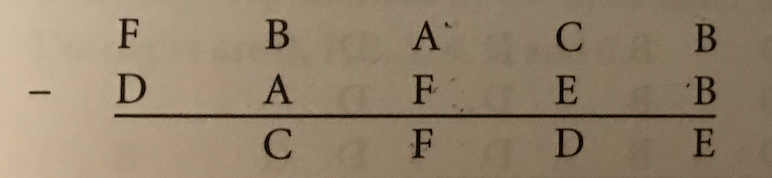12 May 2019

# Sunday morning puzzle

Here is a puzzle we were solving this morning.

Each letter stands for a digit. Those digits are 0,1,2,3,4,5

One twist – The letters below the line do NOT match the letters above the line in terms of the digits they represent. In fact, the digit represented by a letter above the line is separated from the digit represented by the same letter below the line by 1. So if C below the line is 2, then C above the line has to be either 1 or 3.

Can you solve the following subtraction? Send me a message with the answer.Posted May 12, 2019 by rajibroy in category "Puzzles

1.By S on
2.By rajibroy (Post author) on